# QlikView App Dev

Discussion Board for collaboration related to QlikView App Development.

Announcements
Join us at the Cloud Data and Analytics Tour! REGISTER TODAY
cancel
Showing results for
Search instead for
Did you mean:Contributor

## Pivot table Value Wrongly calculates Interval

Hi,

I am trying to post a Sum of HOURS field in a pivot table & the HOURS field has values like HH:MM (60 minutes format)

But When i try to sum this in a Pivot table, The result value is shown as HH:MM (100 minutes format)

For example, sum of entries like 2.30 and 2.40 is giving me 4.80 but i expected it to be 5.10

Can you help me with correct format ?.

I tried to use interval function like =interval(Sum(HOURS)/24,'h.mm')) , but it's diving total by 24 instead of actual function.

Labels (4)

• ### QlikView 12.2

1 Solution

Accepted Solutions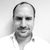Contributor III

Hey

There area three problems.  Your expression is summing the hours before they are converted to a time.  SO it will treat the .3 as a decimal and not a half hour.  The sum needs to sit around the interval#

=Interval(Sum(Interval#(hours, 'h.mm')), 'h.mm')

Secondly, the format is wrong because you have set the format manually in the "Number" tab.  The expression is forced to be Fixed at 2 decimal places, so it converts the number from a time format.  Change it back to expression default.

Finally, your 10.3 will be read as 10 hours and 3 minutes, not 30 which I suspect it should be.  You need to get your data collection to be a bit better, or affect the format of the data so that it properly reflects the time in a correct format.

So AB right now which has 5 sets of 10.3 hours reads as 50.15 hours and not 52.5.  It may be that if there is only one number after the decimal place then you automatically add a 0 to it, but better you get the source data to be a proper time format.

Hey, Please don't forget to add kudos, like or mark as a solution if my reply has helped you at all! 🙂
15 RepliesMVP

Try this instead

``=Interval(Sum(Interval#(HOURS, 'h.mm')), 'h.mm'))``Contributor
Author

@sunny_talwar  That formula is again doing some division operation instead of formatting.

For example, It's converting 395.83 which is total Sum to 16.49

OR

395.83/24 = 16.49MVP

Not sure I understand... it is converting 395.83 to 16.49? But hard-coding it gives me a different value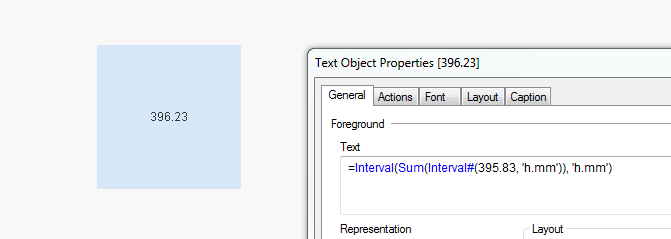Contributor
Author

Yes. It's dividing Sum by 24. Not sure why. May be it has something to do with Pivot table funcationality.

=Sum(HOURS) it shows me 395.83

But your formula is giving me 16.49MVP

I don't know why you see 16.49... did you remove the division by 24? My expression didn't divide by 24... but you original expression did...

`=Interval(Sum(Interval#(HOURS, 'h.mm')), 'h.mm'))`Contributor
Author

Hi,

I am not doing any division by 24. I am using the same formula as you proposed but it's internally dividing by 24 and producing wrong result.

It's kind of a bug it seems.MVP

Are you able to share a sample where we can see the issue?Contributor
Author

Please see the difference i get when i apply the formula.

If i just do a Sum, i get 50.** but when i apply formula it divides by 24 to give me 2.**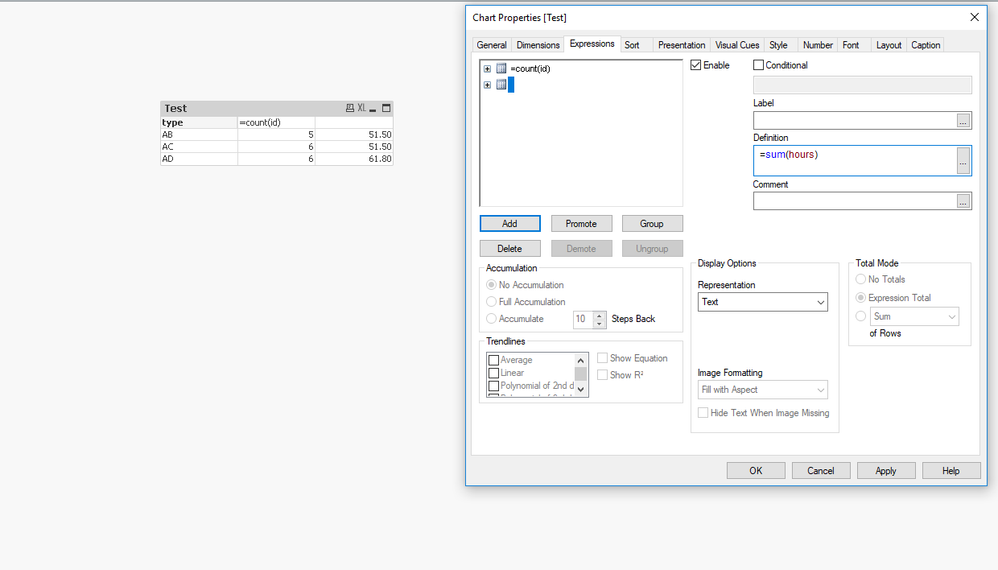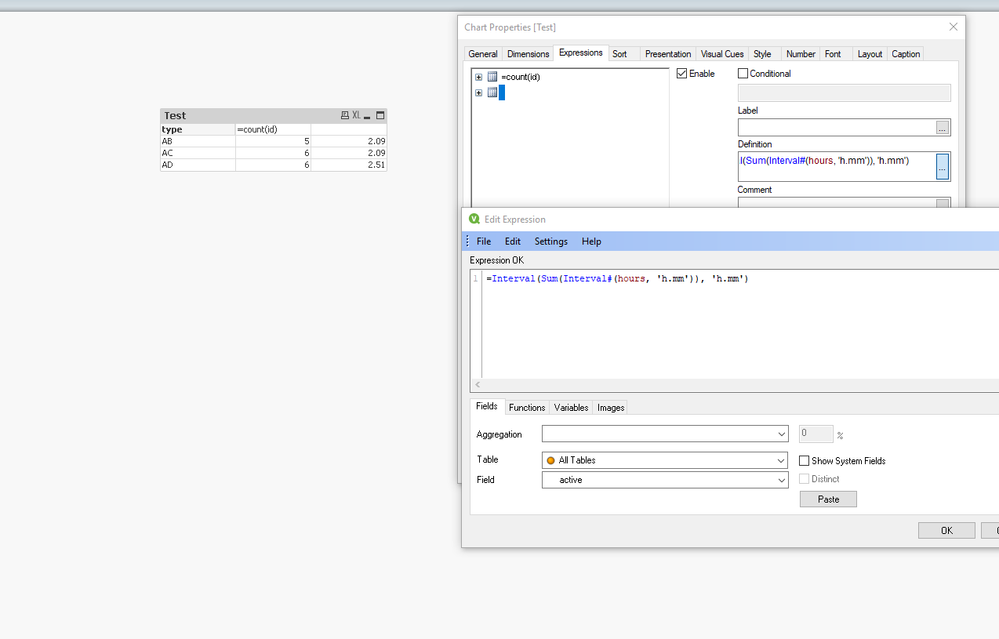Contributor III

Are the numbers you are using decimals and not a time format, so .5 will not be interpreted as 30 minutes.

Turn it into a round number with minutes as the denominator.

=Interval(sum(Interval#(Hours*60,'mm')),'hh:mm')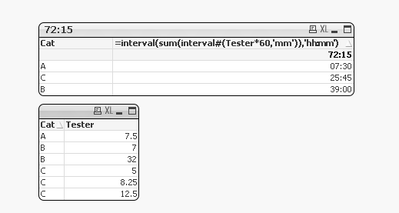Otherwise can you post the background data please as a table.

Hey, Please don't forget to add kudos, like or mark as a solution if my reply has helped you at all! 🙂